# Semi-simple matrix

(diff) ← Older revision | Latest revision (diff) | Newer revision → (diff)
A square matrix over a fieldsimilar to a matrix of the form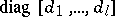, where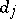is a matrix overwhose characteristic polynomial is irreducible in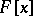,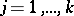(cf. Irreducible polynomial). For a matrixover a field, the following three statements are equivalent: 1)is semi-simple; 2) the minimum polynomial ofhas no multiple factors in; and 3) the algebra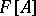is semi-simple (cf. Semi-simple algebra).
Ifis a perfect field, then a semi-simple matrix overis similar to a diagonal matrix over a certain extension of. For any square matrixover a perfect field there is a unique representation in the form, where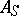is a semi-simple matrix,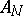is nilpotent and; the matricesandbelong to the algebra.